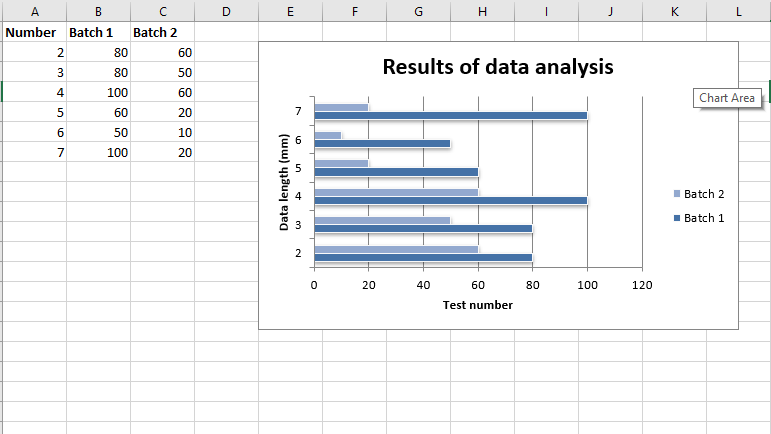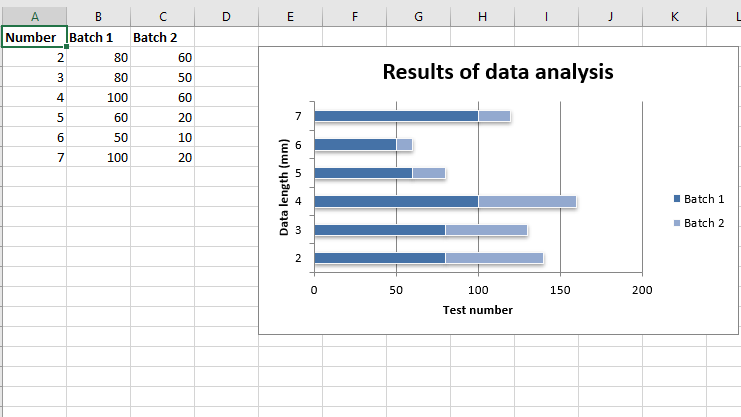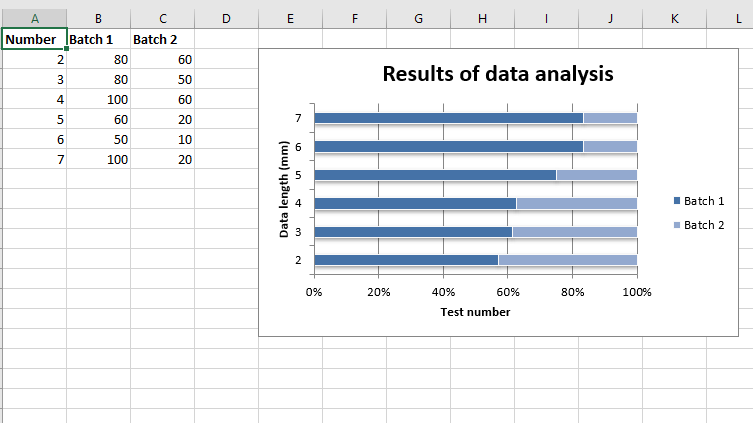# Python | Plotting bar charts in excel sheet using XlsxWriter module

• Last Updated : 19 May, 2021

Prerequisite: Create and Write on an excel file.
XlsxWriter is a Python library using which one can perform multiple operations on excel files like creating, writing, arithmetic operations and plotting graphs. Let’s see how to plot different type of Bar charts using realtime data.
Charts are composed of at least one series of one or more data points. Series themselves are comprised of references to cell ranges.
For plotting the charts on an excel sheet, firstly, create chart object of specific chart type( i.e Bar, Stacked Bar, Percent Stacked Bar chart etc.). After creating chart objects, insert data in it and lastly, add that chart object in the sheet object.
Code #1 : Plot the simple Bar Chart.
For plotting the simple Bar chart on an excel sheet, use add_chart() method with type ‘bar’ keyword argument of a workbook object.

## Python3

 `# import xlsxwriter module``import` `xlsxwriter` `# Workbook() takes one, non-optional, argument ``# which is the filename that we want to create.``workbook ``=` `xlsxwriter.Workbook(``'chart_bar.xlsx'``)` `# The workbook object is then used to add new ``# worksheet via the add_worksheet() method.``worksheet ``=` `workbook.add_worksheet()` `# Create a new Format object to formats cells``# in worksheets using add_format() method .` `# here we create bold format object .``bold ``=` `workbook.add_format({``'bold'``: ``1``})` `# create a data list .``headings ``=` `[``'Number'``, ``'Batch 1'``, ``'Batch 2'``]` `data ``=` `[``    ``[``2``, ``3``, ``4``, ``5``, ``6``, ``7``],``    ``[``80``, ``80``, ``100``, ``60``, ``50``, ``100``],``    ``[``60``, ``50``, ``60``, ``20``, ``10``, ``20``],``]` `# Write a row of data starting from 'A1'``# with bold format .``worksheet.write_row(``'A1'``, headings, bold)` `# Write a column of data starting from``# 'A2', 'B2', 'C2' respectively .``worksheet.write_column(``'A2'``, data[``0``])``worksheet.write_column(``'B2'``, data[``1``])``worksheet.write_column(``'C2'``, data[``2``])` `# Create a chart object that can be added``# to a worksheet using add_chart() method.` `# here we create a bar chart object .``chart1 ``=` `workbook.add_chart({``'type'``: ``'bar'``})` `# Add a data series to a chart``# using add_series method.` `# Configure the first series.``# = Sheet1 !\$A\$1 is equivalent to ['Sheet1', 0, 0].``chart1.add_series({``    ``'name'``:       ``'= Sheet1 !\$B\$1'``,``    ``'categories'``: ``'= Sheet1 !\$A\$2:\$A\$7'``,``    ``'values'``:     ``'= Sheet1 !\$B\$2:\$B\$7'``,``})` `# Configure a second series.``# Note use of alternative syntax to define ranges.``# [sheetname, first_row, first_col, last_row, last_col].``chart1.add_series({``    ``'name'``:       [``'Sheet1'``, ``0``, ``2``],``    ``'categories'``: [``'Sheet1'``, ``1``, ``0``, ``6``, ``0``],``    ``'values'``:     [``'Sheet1'``, ``1``, ``2``, ``6``, ``2``],``})` `# Add a chart title``chart1.set_title ({``'name'``: ``'Results of data analysis'``})` `# Add x-axis label``chart1.set_x_axis({``'name'``: ``'Test number'``})` `# Add y-axis label``chart1.set_y_axis({``'name'``: ``'Data length (mm)'``})` `# Set an Excel chart style.``chart1.set_style(``11``)` `# add chart to the worksheet``# the top-left corner of a chart``# is anchored to cell E2 .``worksheet.insert_chart(``'E2'``, chart1)` `# Finally, close the Excel file``# via the close() method.``workbook.close()`

Output:Code #2 : Plot the Stacked Bar Chart.
For plotting the Stacked Bar chart on an excel sheet, use add_chart() method with type ‘bar’ and subtype ‘stacked’ keyword argument of a workbook object.

## Python3

 `# import xlsxwriter module``import` `xlsxwriter` `# Workbook() takes one, non-optional, argument ``# which is the filename that we want to create.``workbook ``=` `xlsxwriter.Workbook(``'chart_bar2.xlsx'``)` `# The workbook object is then used to add new ``# worksheet via the add_worksheet() method.``worksheet ``=` `workbook.add_worksheet()` `# Create a new Format object to formats cells``# in worksheets using add_format() method .` `# here we create bold format object .``bold ``=` `workbook.add_format({``'bold'``: ``1``})` `# create a data list .``headings ``=` `[``'Number'``, ``'Batch 1'``, ``'Batch 2'``]` `data ``=` `[``    ``[``2``, ``3``, ``4``, ``5``, ``6``, ``7``],``    ``[``80``, ``80``, ``100``, ``60``, ``50``, ``100``],``    ``[``60``, ``50``, ``60``, ``20``, ``10``, ``20``],``]` `# Write a row of data starting from 'A1'``# with bold format .``worksheet.write_row(``'A1'``, headings, bold)` `# Write a column of data starting from``# 'A2', 'B2', 'C2' respectively .``worksheet.write_column(``'A2'``, data[``0``])``worksheet.write_column(``'B2'``, data[``1``])``worksheet.write_column(``'C2'``, data[``2``])` `# Create a chart object that can be added``# to a worksheet using add_chart() method.` `# here we create a stacked bar chart object .``chart1 ``=` `workbook.add_chart({``'type'``: ``'bar'``, ``'subtype'``: ``'stacked'``})` `# Add a data series to a chart``# using add_series method.` `# Configure the first series.``# = Sheet1 !\$A\$1 is equivalent to ['Sheet1', 0, 0].``chart1.add_series({``    ``'name'``:       ``'= Sheet1 !\$B\$1'``,``    ``'categories'``: ``'= Sheet1 !\$A\$2:\$A\$7'``,``    ``'values'``:     ``'= Sheet1 !\$B\$2:\$B\$7'``,``})` `# Configure a second series.``# Note use of alternative syntax to define ranges.``# [sheetname, first_row, first_col, last_row, last_col].``chart1.add_series({``    ``'name'``:       [``'Sheet1'``, ``0``, ``2``],``    ``'categories'``: [``'Sheet1'``, ``1``, ``0``, ``6``, ``0``],``    ``'values'``:     [``'Sheet1'``, ``1``, ``2``, ``6``, ``2``],``})` `# Add a chart title``chart1.set_title ({``'name'``: ``'Results of data analysis'``})` `# Add x-axis label``chart1.set_x_axis({``'name'``: ``'Test number'``})` `# Add y-axis label``chart1.set_y_axis({``'name'``: ``'Data length (mm)'``})` `# Set an Excel chart style.``chart1.set_style(``11``)` `# add chart to the worksheet``# the top-left corner of a chart``# is anchored to cell E2 .``worksheet.insert_chart(``'E2'``, chart1)` `# Finally, close the Excel file``# via the close() method.``workbook.close()`

Output:Code #3 : Plot the Percent Stacked bar Chart.
For plotting the Percent Stacked Bar chart on an excel sheet, use add_chart() method with type ‘bar’ and subtype ‘percent_stacked’ keyword argument of a workbook object.

## Python3

 `# import xlsxwriter module``import` `xlsxwriter` `# Workbook() takes one, non-optional, argument ``# which is the filename that we want to create.``workbook ``=` `xlsxwriter.Workbook(``'chart_bar3.xlsx'``)` `# The workbook object is then used to add new ``# worksheet via the add_worksheet() method.``worksheet ``=` `workbook.add_worksheet()` `# Create a new Format object to formats cells``# in worksheets using add_format() method .` `# here we create bold format object .``bold ``=` `workbook.add_format({``'bold'``: ``1``})` `# create a data list .``headings ``=` `[``'Number'``, ``'Batch 1'``, ``'Batch 2'``]` `data ``=` `[``    ``[``2``, ``3``, ``4``, ``5``, ``6``, ``7``],``    ``[``80``, ``80``, ``100``, ``60``, ``50``, ``100``],``    ``[``60``, ``50``, ``60``, ``20``, ``10``, ``20``],``]` `# Write a row of data starting from 'A1'``# with bold format .``worksheet.write_row(``'A1'``, headings, bold)` `# Write a column of data starting from``# 'A2', 'B2', 'C2' respectively .``worksheet.write_column(``'A2'``, data[``0``])``worksheet.write_column(``'B2'``, data[``1``])``worksheet.write_column(``'C2'``, data[``2``])` `# Create a chart object that can be added``# to a worksheet using add_chart() method.` `# here we create a percent stacked bar chart object .``chart1 ``=` `workbook.add_chart({``'type'``: ``'bar'``, ``'subtype'``: ``'percent_stacked'``})` `# Add a data series to a chart``# using add_series method.` `# Configure the first series.``# = Sheet1 !\$A\$1 is equivalent to ['Sheet1', 0, 0].``chart1.add_series({``    ``'name'``:       ``'= Sheet1 !\$B\$1'``,``    ``'categories'``: ``'= Sheet1 !\$A\$2:\$A\$7'``,``    ``'values'``:     ``'= Sheet1 !\$B\$2:\$B\$7'``,``})` `# Configure a second series.``# Note use of alternative syntax to define ranges.``# [sheetname, first_row, first_col, last_row, last_col].``chart1.add_series({``    ``'name'``:       [``'Sheet1'``, ``0``, ``2``],``    ``'categories'``: [``'Sheet1'``, ``1``, ``0``, ``6``, ``0``],``    ``'values'``:     [``'Sheet1'``, ``1``, ``2``, ``6``, ``2``],``})` `# Add a chart title``chart1.set_title ({``'name'``: ``'Results of data analysis'``})` `# Add x-axis label``chart1.set_x_axis({``'name'``: ``'Test number'``})` `# Add y-axis label``chart1.set_y_axis({``'name'``: ``'Data length (mm)'``})` `# Set an Excel chart style.``chart1.set_style(``11``)` `# add chart to the worksheet``# the top-left corner of a chart``# is anchored to cell E2 .``worksheet.insert_chart(``'E2'``, chart1)` `# Finally, close the Excel file``# via the close() method.``workbook.close()`

Output:My Personal Notes arrow_drop_up